HOME > Master's Program > Department of Mathematical and Physical Sciences > Mathematics Course

# Master's Program in Mathematics Course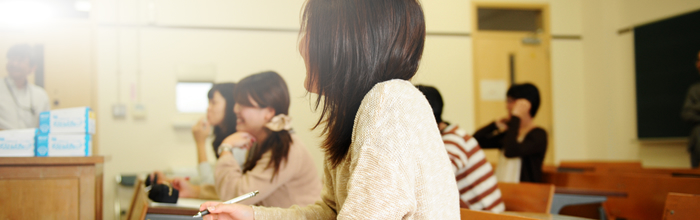Mathematics is the foundation of natural sciences and is applied in various facets of human life. Master course students study analysis of various natural and social phenomena, and construction of mathematical theory by searching the mathematical structures lying in this analysis. Students can learn mathematical capabilities and knowledge through these types of research.

### Faculty and Research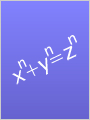Yumiko Umegaki
Associate Professor
Laboratory
Number Theory
Analytic number theory
Keywords : Automorphic L-functions, Zeros, Special values, Analytic rank of elliptic curveTakeo Okazaki
Associate Professor
Laboratory
Algebra
Number theory and varieties
Keywords : Automorphic forms and representations, Modular varieties, L-function, New Forms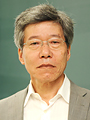Fujio Kako
Professor
Computer Algebra
Algorithm for approximate algebraic computationsMinyo Katagiri
Associate Professor
Laboratory
Geometry
Geometric variational problems; Topological graph theory
Keywords : map coloring, graph polynomial, categorification, cohomology group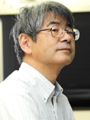Tsuyoshi Kobayashi
Professor
Topology
Three-manifold topology; Geometry of knots and links
Keywords : 3-manifold, Knots and Links, Mixing fluids, Origami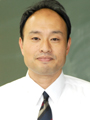Masato Shinoda
Professor
Probability
Probabilistic models of statistical mechanics
Keywords : discrete probabilistic models, fractal sets, mathematical games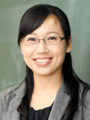Yeonhee Jang
Assistant Professor
Topology
Three-manifold topology, knot theory
Keywords : Knots, bridge splittings, 3-manifolds, Heegaard splittingsTomoko Takemura
Assistant Professor
Laboratory
Probability
Probability and stochastic analysis
Keywords : Diffusion process, limit theorem, skew product, harmonic transform, Dirichlet FormShuichiro Tsunoda
Professor
Laboratory
Algebra
Unified theory of algebra, geometry, and analysisJunichi Matsuzawa
Professor
Laboratory
Algebraic Geometry
Representation theory
Keywords : Lie Groups , Lie Algebras, Weyl Groups, Root Systems , Algebraic SurfacesHiroko Murai
Assistant Professor
Laboratory
Topology
Knot theory, 3-Manifold topology, and foliations
Keywords : Knots and links, Closed curves on surfaces, Categorification of knot or graph polynomials, Geometry in OrigamiShinya Moritoh
Professor
Laboratory
Analysis
Fourier analysis, wavelet analysis, and function spaces
Keywords : Fourier, wavelet, function space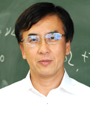Taku Yanagisawa
Professor
Laboratory
Nonlinear Analysis
Nonlinear PDE and fluid mechanics
Keywords : Nonlinear partial differential equations, Boundary value problems, Fluid dynamics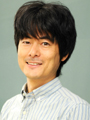Yasushi Yamashita
Professor
Hyperbolic Geometry
Study on hyperbolic structures of low-dimensional manifolds
Keywords : Topology, hyperbolic geometry, visuallization